# Information uncertainty related to marked random times and optimal investment

## Abstract

### ■■■

We study an optimal investment problem under default risk where related information such as loss or recovery at default is considered as an exogenous random mark added at default time. Two types of agents who have different levels of information are considered. We first make precise the insider’s information flow by using the theory of enlargement of filtrations and then obtain explicit logarithmic utility maximization results to compare optimal wealth for the insider and the ordinary agent.

## Introduction

The optimization problem in presence of uncertainty on a random time is an important subject in finance and insurance, notably for risk and asset management when it concerns a default event or a catastrophic occurrence. Another related source of risk is the information associated with the random time concerning resulting payments, the price impact, the loss given default or the recovery rate, etc. Measuring these random quantities is, in general, difficult since the relevant information on the underlying firm is often not accessible to investors on the market. For example, in the credit risk analysis, modelling the recovery rate is a subtle task (see, e.g. Duffie and Singleton (2003) Section 6, Bakshi et al. (2006), and Guo et al. (2009)).

In this paper, we study the optimal investment problem with a random time and consider the information revealed at the random time as an exogenous factor of risk. We suppose that all investors on the market can observe the arrival of the random time, such as the occurrence of a default event. However, for the associated information, such as the recovery rate, there are two types of investors: the first is an informed insider and the second is an ordinary investor. For example, the insider has private information on the loss or recovery value of a distressed firm at the default time, but the ordinary investor must wait for the legitimate procedure to be finished to know the result. Both investors aim at maximizing the expected utility from the terminal wealth and each of them will determine their investment strategy based on the corresponding information set. Following Amendinger et al. (1998, 2003), we will compare the optimization results and deduce the additional gain of the insider.

Let the financial market be described by a probability space $$(\Omega,\mathcal A,\mathbb {P})$$ equipped with a reference filtration $$\mathbb {F}=(\mathcal {F}_t)_{t\geq 0}$$ which satisfies the usual conditions. In the literature, the theory of enlargements of filtrations provides essential tools for the modelling of different information flows. In general, the observation of a random time, in particular a default time, is modelled by the progressive enlargement of filtration, as proposed by Elliott et al. (2000) and Bielecki and Rutkowski (2002). The knowledge of insider information is usually studied by using the initial enlargement of filtration as in Amendinger et al. (1998, 2003) and Grorud and Pontier (1998). In this paper, we suppose that the filtration $$\mathbb {F}$$ represents the market information known by all investors, including the default information. Let τ be an $$\mathbb {F}$$-stopping time which represents the default time. The information flow associated with τ is modelled by a random variable G on $$(\Omega,\mathcal A)$$ valued in a measurable space $$(E,\mathcal {E})$$. In the classic setting of insider information, G is added to $$\mathbb {F}$$ at the initial time t=0, while in our model, the information is added punctually at the random time τ. Therefore, we need to specify the corresponding filtration. Let the insider’s filtration $$\mathbb {G}=(\mathcal {G}_t)_{t\geq 0}$$ be a punctual enlargement of $$\mathbb {F}$$ by adding the information of G at the random time τ. In other words, $$\mathbb {G}$$ is the smallest filtration which contains $$\mathbb {F}$$ and such that the random variable G is $$\mathcal {G}_{\tau }$$-measurable. This provides a new type of enlargement of filtrations which is an extension of the classical initial enlargement. In the literature, other generalizations of enlargement have also been considered such as in Kchia et al. (2013) and Kchia and Protter (2015) where the authors study extensions of progressive enlargement.

We shall make precise the adapted and predictable processes in the filtration $$\mathbb {G}$$ that we define in order to describe investment strategy and wealth processes. As usual in the asymmetric information literature, we suppose the hypothesis that the $$\mathbb {F}$$-conditional law of G is equivalent and hence admits a positive density with respect to its probability law. By adapting arguments in Föllmer and Imkeller (1993) and in Grorud and Pontier (1998), we deduce the insider martingale measure $$\mathbb {Q}$$ which plays an important role in the study of (semi)martingale processes in the filtration $$\mathbb {G}$$. Our main mathematical result is to give the decomposition formula of an $$\mathbb {F}$$-martingale as a semimartingale in $$\mathbb {G}$$, which gives a positive answer to the Jacod’s (H’)-hypothesis.

In the optimization problem with random default times, it is often supposed that the random time satisfies the intensity hypothesis (e.g., Lim and Quenez (2011) and Kharroubi et al. (2013)) or the density hypothesis (e.g., Blanchet-Scalliet et al. (2008), Jeanblanc et al. (2015), and Jiao et al. (2013)), so that it is a totally inaccessible stopping time in the market filtration. In particular, in Jiao et al. (2013), we consider marked random times where the random mark represents the loss at default and we suppose that the vector of default time and mark admits a conditional density. In this paper, the random time τ we consider does not necessarily satisfy the intensity nor the density hypothesis: it is a general stopping time in $$\mathbb {F}$$ and may also contain a predictable part. Following the approach of Amendinger et al. (1998), we obtain the optimal strategy and wealth for the two types of investors with a logarithmic utility function and deduce, thanks to the decomposition we get before, the additional gain due to the extra information. As a concrete case, we consider a hybrid default model similar as in Campi et al. (2009) where the filtration $$\mathbb {F}$$ is generated by a Brownian motion and a Poisson process, the default time is the minimum of two random times: the first hitting time of a Brownian diffusion and the first jump time of the Poisson process. The noticeable fact is that the previous characterization of the optimal expected wealth allows to derive an explicit formula for the additional expected utility.

The rest of the paper is organized as following. We model in Section 2 the filtration which represents the default time together with the random mark and study its theoretical properties. Section 3 focuses on the logarithmic utility optimization problem for the insider and compares the result with the case for ordinary investor. In Section 4, we present the optimization results for an explicit hybrid default model. Section 5 concludes the paper.

## Model framework

In this section, we present our model setup. In particular, we study the enlarged filtration including a random mark, which is an extension of the classical initial enlargement of filtrations. To the best of our knowledge such an enlargement has not been considered before.

### The enlarged filtration and martingale processes

Let $$(\Omega,\mathcal {A},\mathbb {P})$$ be a probability space equipped with a filtration $$\mathbb {F}=(\mathcal {F}_t)_{t\geq 0}$$ which satisfies the usual conditions and τ be an $$\mathbb {F}$$-stopping time. Let G be a random variable valued in a measurable space $$(E,\mathcal {E})$$ and $$\mathbb {G}=(\mathcal {G}_t)_{t\geq 0}$$ be the smallest filtration containing $$\mathbb {F}$$ such that G is $$\mathcal {G}_{\tau }$$-measurable. By definition, one has

$$\forall\,t\in\mathbb R_+,\quad\mathcal{G}_t=\mathcal{F}_{t}\vee\sigma\Big(\big\{A\cap\{\tau\leq s \}\,|\,A\in\sigma(G),\; s\leq t\big\}\Big).$$
(2.1)

In particular, similarly to Jeulin (1980) (see also Callegaro et al. (2013)), a stochastic process Z is $$\mathbb {G}$$-adapted if and only if it can be written in the form(2.2)

where Y is an $$\mathbb {F}$$-adapted process and Y(·) is an $$\mathbb {F}\otimes \mathcal {E}$$-adapted process on Ω×E, where $$\mathbb {F}\otimes \mathcal {E}$$ denotes the filtration $$(\mathcal {F}_{t}\otimes \mathcal {E})_{t\geq 0}$$. The following proposition characterizes the $$\mathbb {G}$$-predictable processes. The proof combines the techniques from those of Lemmata 3.13 and 4.4 in Jeulin (1980) and is postponed to Appendix.

### Proposition 2.1

Let $$\mathcal {P}(\mathbb {F})$$ be the predictable σ-algebra of the filtration $$\mathbb {F}$$. A $$\mathbb {G}$$-adapted process Z is $$\mathbb {G}$$-predictable if and only if it can be written in the form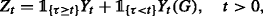(2.3)

where Y is an $$\mathbb F$$-predictable process and Y(·)is a $$\mathcal {P}(\mathbb {F})\otimes \mathcal {E}$$-measurable function.

We study the martingale processes in the filtrations $$\mathbb {F}$$ and $$\mathbb {G}$$. One basic martingale in $$\mathbb {F}$$ is related to the random time τ. Let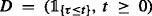be the indicator process of the $$\mathbb {F}$$-stopping time τ. Recall that the $$\mathbb {F}$$-compensator process Λ of τ is the $$\mathbb {F}$$-predictable increasing process Λ such that the process N defined by N:=DΛ is an $$\mathbb {F}$$-martingale. In particular, if τ is a predictable $$\mathbb {F}$$-stopping time, then Λ coincides with D.

To study $$\mathbb {G}$$-martingales, we assume the following hypothesis for the random variable G with respect to the filtration $$\mathbb {F}$$ (c.f. Grorud and Pontier (1998) in the initial enlargement setting, see also Jacod (1985) for comparison).

### Assumption 2.2

For any t≥0, the $$\mathcal {F}_{t}$$-conditional law of G is equivalent to the probability law η of G, i.e., $$\mathbb {P}(G\in \cdot |\mathcal {F}_t)\sim \eta (\cdot)$$, a.s. Moreover, we denote by p t (·) the conditional density

$$\mathbb{P}(G\in dx|\mathcal{F}_t)=p_{t}(x)\eta(dx),\quad a.s.$$
(2.4)

As pointed out in Lemma 1.8 of Jacod (1985), we can choose a version of the conditional probability density p(·), such that p t (·) is $$\mathcal {F}_{t}\otimes \mathcal {E}$$-measurable for any t≥0 and that (p t (x), t≥0) is a positive càdlàg $$(\mathbb {F},\mathbb {P})$$-martingale for any xE. In the following, we will fix such a version of the conditional density.

### Remark 2.3

We assume the hypothesis of Jacod which is widely adopted in the study of initial and progressive enlargements of filtrations. Compared to the standard initial enlargement of $$\mathbb {F}$$ by G, the information of the random variable G is added at a random time τ but not at the initial time; compared to the progressive enlargement, the random variable added here is the associated information G instead of the random time τ. In particular, the behavior of $$\mathbb {G}$$-martingales is quite different from the classic setting, and worth it to be examined in detail.

Similarly to Föllmer and Imkeller (1993) and Grorud and Pontier (1998), we introduce the insider martingale measure $$\mathbb {Q}$$ which will be useful in the remainder of the paper.

### Proposition 2.4

For any t≥0, there exists a unique probability measure $$\mathbb {Q}$$ on $$\mathcal {F}_{t}\vee \sigma (G)$$ which verifies the following conditions:

1. (1)

the probability measures $$\mathbb {Q}$$ and $$\mathbb {P}$$ are equivalent;

2. (2)

$$\mathbb {Q}$$ coincides with $$\mathbb {P}$$ on $$\mathbb {F}$$ and on σ(G);

3. (3)

G is independent of $$\mathbb {F}$$ under the probability $$\mathbb {Q}$$.

Moreover, the Radon-Nikodym density L t of $$\mathbb {Q}$$ with respect to $$\mathbb {P}$$ on $$\mathcal {G}_{t}$$ is given by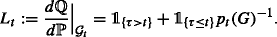(2.5)

### Proof

Let $$\mathbb {Q}$$ be defined by

$$\frac{d\mathbb{Q}}{d\mathbb{P}}\Big|_{\mathcal{F}_{t}\vee\sigma(G)}={p_{t}(G)^{-1}},\quad t\geq 0.$$

Since $$(\mathcal {F}_{t}\vee \sigma (G))_{t\geq 0}$$ is the initial enlargement of $$\mathbb {F}$$ by G, we obtain by Föllmer and Imkeller (1993) and Grorud and Pontier (1998) that $$\mathbb {Q}$$ is the unique equivalent probability measure on $$\mathcal {F}_{t}\vee \sigma (G)$$ which satisfies conditions (1)–(3). Moreover, the Radon-Nikodym density $$d\mathbb {Q}/d\mathbb {P}$$ on $$\mathcal {G}_{t}$$ is given byLet Z t be a bounded $$\mathcal {G}_{t}$$-measurable random variable. By the decomposed form (2.2), we obtain that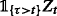is $$\mathcal {F}_{t}$$-measurable. Hence,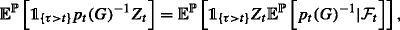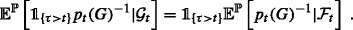Moreover, we have

$$\mathbb{E}^{\mathbb{P}}\left[p_{t}(G)^{-1}|\mathcal{F}_{t}\right] \left. =\frac{d\mathbb{Q}}{d\mathbb{P}}\right|_{\mathcal{F}_t}=1 \quad\text{a.s.}$$

since $$\mathbb {P}$$ and $$\mathbb {Q}$$ coincide on $$\mathbb {F}$$. Therefore, we obtain (2.5). □

In the above Proposition, the probability measure $$\mathbb {Q}$$ depends on the time t since it is defined on the σ-algebra $$\mathcal {F}_{t}\vee \sigma (G)$$. However, the unicity of the equivalent probability measure shows that, if for any t≥0 we denote by $$\mathbb {Q}_{t}$$ the probability measure on $$\mathcal {F}_{t}\vee \sigma (G)$$ which satisfies the conditions (1)-(3) of the proposition, then for 0≤st the restriction of $$\mathbb {Q}_{t}$$ on $$\mathcal {F}_{s}\vee \sigma (G)$$ coincides with $$\mathbb {Q}_{s}$$. This observation allows us to use just $$\mathbb {Q}$$ to denote the probability on $$\bigcup _{t\geq 0}(\mathcal {F}_{t}\vee \sigma (G))$$ which coincides with $$\mathbb {Q}_{t}$$ on $$\mathcal {F}_{t}\vee \sigma (G)$$.

The following proposition shows that the filtration $$\mathbb {G}$$ also satisfies the usual conditions under the $$\mathbb {F}$$-density hypothesis on the random variable G. The idea follows Amendinger (2000, Proposition 3.3).

### Proposition 2.5

Under Assumption 2.2, the enlarged filtration $$\mathbb {G}$$ is right continuous.

### Proof

The statement does not involve the underlying probability measure. Hence, we may assume without loss of generality (by Proposition 2.4) that G is independent of $$\mathbb {F}$$ under the probability $$\mathbb {P}$$. Let $$t\geqslant 0$$ and ε>0. Let Xt+ε be a bounded $$\mathcal {G}_{t+\varepsilon }$$-measurable random variable. We write it in the formwhere Yt+ε and Yt+ε(·) are, respectively, bounded $$\mathcal {F}_{t+\varepsilon }$$-measurable and $$\mathcal {F}_{t+\varepsilon }\otimes \mathcal {E}$$-measurable functions. Then for δ(0,ε), by the independence between G and $$\mathbb {F}$$ one haswhere η is the probability law of G. Since the filtration $$\mathbb {F}$$ satisfies the usual conditions, any $$\mathbb {F}$$-martingale admits a càdlàg version. Therefore, by taking a suitable version of the expectations $$\mathbb {E}^{\mathbb {P}}\left [X_{t+\varepsilon }\,|\,\mathcal {F}_{t+\delta }\right ]$$, we haveIn particular, if X is a bounded $$\mathcal {G}_{t+}:=\bigcap _{\varepsilon >0}\mathcal {G}_{t+\varepsilon }$$-measurable random variable, then one has $$\mathbb {E}^{\mathbb {P}}[X\,|\,\mathcal {G}_{t}]=X$$ almost surely. Hence $$\mathcal {G}_{t+}=\mathcal {G}_{t}$$. □

Under the probability measure $$\mathbb {Q}$$, the random variable G is independent of $$\mathbb {F}$$. This observation leads to the following characterization of $$(\mathbb {G},\mathbb {Q})$$-(local)-martingales. In the particular case where τ=0, we recover the classic result on initial enlargement of filtrations. In the sequel, we denote by $$\langle.,.\rangle _{t}^{\mathbb {F},\mathbb {P}}$$ the angle bracket with respect to $$\mathbb {F}$$ under $$\mathbb {P}$$ and we define the process I(Y(.)) by

$$I_{t}(Y(.)) = \int_{E}\left(\int_{]0,t]}Y_{u-}(x)\,d\Lambda_u+\langle N,Y(x)\rangle_{t}^{\mathbb{F},\mathbb{P}}\right)\eta(dx), \quad t\geq 0$$
(2.6)

for a càdlàg $$\mathbb {F}\otimes \mathcal {E}$$-adapted process Y(·).

### Proposition 2.6

Letbe a $$\mathbb {G}$$-adapted process. We assume that

1. (1)

Y(·) is an $$\mathbb {F}\otimes \mathcal {E}$$-adapted process such that Y(x) is an $$(\mathbb {F},\mathbb {P})$$-square-integrable martingale for any xE (resp., an $$(\mathbb {F},\mathbb {P})$$-locally square-integrable martingale with a common localizing stopping time sequence independent of x),

2. (2)

the processis well defined and is an $$(\mathbb {F},\mathbb {P})$$-martingale (resp., an $$(\mathbb {F},\mathbb {P})$$-local martingale).

Then, the process Z is a $$(\mathbb {G},\mathbb {Q})$$-martingale (resp., a $$(\mathbb {G},\mathbb {Q})$$-local martingale).

### Proof

We can reduce the local martingale case to the martingale case by taking a sequence of $$\mathbb {F}$$-stopping times which localizes the processes appearing in conditions (1) and (2). Therefore, we only treat the martingale case. Note that since N and Y(x) are square integrable (c.f. Chapitre VII (15.1) in Dellacherie and Meyer (1980) for the square integrability of N), $$NY(x)-\langle N,Y(x)\rangle ^{\mathbb {F},\mathbb {P}}$$ is an $$(\mathbb {F},\mathbb {P})$$-martingale by Chapter I, Theorem 4.2 in Jacod and Shiryaev (2003).

For ts≥0, one has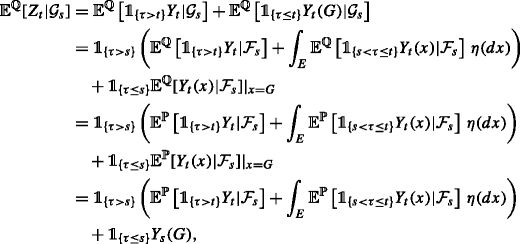(2.7)

where the second equality comes from the fact that G is independent of $$\mathbb {F}$$ under the probability $$\mathbb {Q}$$ and that η coincides with the $$\mathbb {Q}$$-probability law of G, and the third equality comes from the fact that the probability measures $$\mathbb {P}$$ and $$\mathbb {Q}$$ coincide on the filtration $$\mathbb {F}$$.

Since Y(x) is an $$(\mathbb {F},\mathbb {P})$$-martingale, one has(2.8)

where the last equality comes from the fact that $$NY(x)-\langle N,Y(x)\rangle ^{\mathbb {F},\mathbb {P}}$$ is an $$(\mathbb {F},\mathbb {P})$$-martingale. Moreover, since Y(x) is an $$(\mathbb {F},\mathbb {P})$$-martingale, its predictable projection is Y(x) (see Chapter I, Corollary 2.31 in Jacod and Shiryaev (2003)), and hence

$$\mathbb{E}^{\mathbb{P}}[\Lambda_tY_{t}(x)-\Lambda_sY_{s}(x)\,|\,\mathcal{F}_{s}]=\mathbb{E}\bigg[\int_{(s,t]}Y_{u-}(x)\,d\Lambda_{u}\,\bigg|\,\mathcal{F}_{s}\bigg]$$
(2.9)

since Λ is an integrable increasing process which is $$\mathbb {F}$$-predictable (see Chapter VI, 61 in Dellacherie and Meyer (1980)). Therefore, by (2.7) we obtainThe proposition is thus proved. □

### Corollary 2.7

Let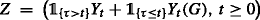be a $$\mathbb {G}$$-adapted process. Then Z is a $$(\mathbb {G},\mathbb {P})$$-martingale (resp., local $$(\mathbb {G},\mathbb {P})$$-martingale) if the following conditions are fulfilled:

1. 1.

for any xE, (Y t (x)p t (x),t≥0) is an $$(\mathbb {F},\mathbb {P})$$-square integrable martingale (resp., a $$(\mathbb {F},\mathbb {P})$$-locally square integrable martingale with a common localizing stopping time sequence);

2. 2.

the processis a $$(\mathbb {F},\mathbb {P})$$-martingale (resp., a local $$(\mathbb {F},\mathbb {P})$$-martingale).

### Proof

By Proposition 2.4, Z is a $$(\mathbb {G},\mathbb {P})$$-(local)-martingale if and only if the process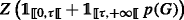is a $$(\mathbb {G},\mathbb {Q})$$-(local)-martingale. Therefore, the assertion results from Proposition 2.6. □

### Proposition 2.8

Let Z be a $$(\mathbb {G},\mathbb {P})$$-martingale on [0,T] such that the processis bounded. Then there exists an $$\mathbb F$$-adapted process Y and an $$\mathbb F\otimes \mathcal E$$-adapted process Y(·) such thatand that the following conditions are fulfilled:

1. (1)

for any xE, (Y t (x)p t (x),t≥0) is a bounded $$(\mathbb {F},\mathbb {P})$$-martingale;

2. (2)

the processis well defined and is an $$(\mathbb {F},\mathbb {P})$$-martingale.

### Proof

Since Z T is a $$\mathcal {G}_{t}$$-measurable random variable, we can write it in the form(2.10)

where Y T is an $$\mathcal {F}_{t}$$-measurable random variable, and Y T (·) is an $$\mathcal {F}_{t}\otimes \mathcal {E}$$-measurable function such that Y T (·)p T (·) is bounded. Similarly to Lemma 1.8 in Jacod (1985), we can construct an $$\mathbb {F}\otimes \mathcal {E}$$-adapted process Y(·) on [0,T] such that Y(x)p(x) is a càdlàg $$(\mathbb {F},\mathbb {P})$$-martingale for any xE. In particular, for t[0,T] one has

$$Y_{t}(x)=\mathbb{E}^{\mathbb{P}}\bigg[\frac{Y_{T}(x)p_{T}(x)}{p_{t}(x)}\bigg|\mathcal{F}_{t}\bigg].$$
(2.11)

We then let, for t[0,T]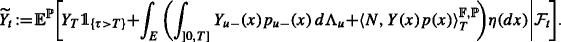(2.12)

Then $$\widetilde Y$$ is an $$(\mathbb {F},\mathbb {P})$$-martingale. For any t[0,T], we let Y t be an $$\mathcal {F}_{t}$$-measurable random variable such thatThis is always possible sincewhere the second equality is obtained by an argument similar to (2.8) and (2.9). We finally show that$$\mathbb {P}$$-a.s. for any t[0,T]. Note that we already have. Therefore it remains to prove that the $$\mathbb G$$-adapted processis a $$(\mathbb {G},\mathbb {P})$$-martingale. This follows from the construction of the processes Y, Y(·) and Corollary 2.7. □

### Remark 2.9

1. (1)

We observe from the proof of the previous proposition that, if Z is a $$(\mathbb {G},\mathbb {P})$$-martingale on [0,T] (without boundedness hypothesis) such that Z T can be written into the form (2.10) with $$Y_{T}(x)p_{T}(x)\in L^{2}(\Omega,\mathcal {F}_{T},\mathbb {P})$$ for any xE, then we can construct the $$\mathbb {F}\otimes \mathcal {E}$$-adapted process Y(·) by using the relation (2.11). Note that for any xE, the process Y(x)p(x) is a square-integrable $$(\mathbb {F},\mathbb {P})$$-martingale. Therefore, the result of Proposition 2.8 remains true provided that the conditional expectation in (2.12) is well defined.

2. (2)

Let Z be a $$(\mathbb {G},\mathbb {P})$$-martingale on [0,T]. In general, the decomposition of Z into the formwith Y being $$\mathbb {F}$$-adapted and Y(·) being $$\mathbb {F}\otimes \mathcal {E}$$-adapted is not unique. Namely, there may exist an $$\mathbb {F}$$-adapted process $$\widetilde {Y}$$ and an $$\mathbb {F}\otimes \mathcal {E}$$-adapted process $$\widetilde Y(\cdot)$$ such that $$\widetilde {Y}$$ is not a version of Y, $$\widetilde {Y}(\cdot)$$ is not a version of Y(·), but we still haveMoreover, although the proof of Proposition 2.8 provides an explicit way to construct the decomposition of the $$(\mathbb {G},\mathbb {P})$$-martingale Z which satisfies the two conditions, in general such decomposition is not unique either.

3. (3)

Concerning the local martingale analogue of Proposition 2.8, the main difficulty is that a local $$(\mathbb {G},\mathbb {P})$$-martingale need not be localized by a sequence of $$\mathbb {F}$$-stopping times. To solve this problem, it is crucial to understand the $$\mathbb {G}$$-stopping times and their relation with $$\mathbb {F}$$-stopping times.

### (H’)-hypothesis and semimartingale decomposition

In this subsection, we prove that under Assumption 2.2, the (H’)-hypothesis, i.e. any $$\mathbb {F}$$-local martingale is a $$\mathbb {G}$$-semimartigale, is satisfied and we give the semimartingale decomposition of an $$\mathbb {F}$$-martingale in $$\mathbb {G}$$.

### Theorem 2.10

We suppose that Assumption 2.2 holds. Let M be an $$(\mathbb {F},\mathbb {P})$$-locally square integrable martingale, then it is a $$(\mathbb {G},\mathbb {P})$$-semimartingale. Let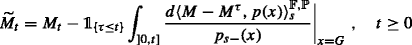and suppose in addition that the process $$\widetilde {M}-M^{\tau }$$ is positive or integrable where $$M^{\tau }=\left (M_{t}^{\tau },t\geq 0\right)$$ is the stopped process with $$M_{t}^{\tau }=M_{t\wedge \tau }$$. Then the process $$\widetilde {M}= \left (\widetilde {M}_{t},t\geq 0\right)$$ is a $$(\mathbb {G},\mathbb {P})$$-local martingale.

### Proof

Let $$\mathbb {I}=\mathbb {F}\vee \sigma (G)$$ be the initial enlargement of the filtration $$\mathbb {F}$$ by σ(G). Clearly, the filtration $$\mathbb {I}$$ is larger than $$\mathbb {G}$$. More precisely, using Lemma 2.4 and 2.5 in Kchia et al. (2013), we get that the filtration $$\mathbb {G}$$ coincides with $$\mathbb {F}$$ before the stopping time τ, and coincides with $$\mathbb {I}$$ after the stopping time τ. We first observe that the stopped process at τ of an $$(\mathbb {F},\mathbb {P})$$-martingale L is a $$(\mathbb {G},\mathbb {P})$$-martingale. In fact, for ts≥0 one hasWe remark that, as shown by Jeulin’s formula, this result holds more generally for any enlargement $$\mathbb {G}$$ which coincides with $$\mathbb {F}$$ before a random time τ.

We now consider the decomposition of M as M=Mτ+(MMτ), where Mτ is the stopped process of M at τ. Since $$\mathbb {G}$$ coincides with $$\mathbb {F}$$ before τ, we obtain by the above argument that Mτ is an $$(\mathbb {G},\mathbb {P})$$-local martingale. Now consider the process Y:=MMτ, which begins at τ. It is also an $$(\mathbb {F},\mathbb {P})$$-local martingale. By Jacod’s decomposition formula (see Theorem 2.1 in Jacod 1985), the process

$$\widetilde{Y}_t=Y_t-\int_{]0,t]}\frac{d\langle Y,p(x)\rangle_{s}^{\mathbb{F},\mathbb{P}}}{p_{s-}(x)}\Big|_{x=G},\quad t\geq 0$$
(2.13)

is an $$(\mathbb {I},\mathbb {P})$$-local martingale. Note that the predictable quadratic variation process $$\langle Y,p(x)\rangle _{s}^{\mathbb {F},\mathbb {P}}$$ vanishes on [ [0,τ] ] since the process Y begins at τ. Hence,

$$\int_{]0,t]}\frac{d\langle Y,p(x)\rangle_s^{\mathbb{F},\mathbb{P}}}{p_{s-}(x)}=\mathbb{1}_{\{\tau\leq t\}}\int_{]0,t]}\frac{d\langle Y,p(x)\rangle_s^{\mathbb{F},\mathbb{P}}}{p_{s-}(x)}.$$

This observation also shows that the process (2.13) is $$\mathbb {G}$$-adapted. From Proposition 1.29 (c) in Aksamit and Jeanblanc (2017) it is a $$(\mathbb {G},\mathbb {P})$$-local martingale. □

### Remark 2.11

1. (1)

In the above theorem, we can weaken Assumption 2.2. Indeed, to apply Jacod’s decomposition formula we only need to assume that the conditional law $$\mathbb {P}(G\in.|\mathcal {F}_t)$$ is absolutely continuous w.r.t. $$\mathbb {P}(G\in.)$$. However, the equivalence assumption is important in the literature on asymmetric information (e.g. Amendinger et al. (1998,2003); Grorud and Pontier (1998)) since it ensures the existence of the decoupling measure (see Proposition 2.4) under which the information variable and the reference filtration are independent.

2. (2)

We present in “Second proof of Theorem 2.10” section which is more computational and longer. However, it provides an explicit way for the construction of the $$\mathbb {G}$$-martingale part and has its own interest. In addition, it allows to remove the positivity or integrability condition on the process $$\widetilde {M}-M^{\tau }$$.

## Logarithmic utility maximization

In this section, we study the optimization problem for two types of investors: an insider and an ordinary agent. We consider a financial market composed of d stocks with discounted prices given by the d-dimensional process X=(X1,…,Xd). This process is observed by both agents and is $$\mathbb {F}$$-adapted. We suppose that each Xi, i=1,…,d, evolves according to the following stochastic differential equation

$$X^{i}_{t} = X_{0}^{i} + \int_{0}^{t} X^{i}_{s-} \left(dM^{i}_{s}+\sum_{j=1}^{d}\alpha^{j}_{s} d \left\langle M^{i}, M^{j} \right\rangle_{s}\right)\;,\quad t\geq 0\;,$$

with $$X^{i}_{0}$$ a positive constant, Mi an $$\mathbb {F}$$-locally square integrable martingale, and α a $$\mathcal {P}(\mathbb {F})$$–measurable process valued in $$\mathbb {R}^{d}$$ such that

$$\mathbb{E}\left[\int_{0}^{T} \alpha^{\top}_{s} d \langle{M} \rangle_{s} \alpha_{s}\right] < +\infty\;.$$
(3.1)

The ordinary agent has access to the information flow given by the filtration $$\mathbb {F}$$, while the information flow of the insider is represented by the filtration $$\mathbb {G}$$. From the practical point of view, when a random event (which can be more general than a default time) arrives, there will often be some extra accompanying information revealed at the random time. Our intuition is to use the random variable G to represent such information. From the mathematical point of view, the filtration G can be viewed as an extension of the classical initial enlargement of filtration where the information is added only at the initial time 0.

The optimization for the ordinary agent is standard (see for example Lim and Quenez (2011)). For the insider, we follow Amendinger et al. (1998,2003) to solve the problem. We first describe the insider’s portfolio in the enlarged filtration $$\mathbb {G}$$. Recall that under Assumption 2.2, the process M is a $$\mathbb {G}$$-semimartingale with canonical decomposition given by Theorem 2.10:

$$M_t = \widetilde{M} _t +\mathbb{1}_{\{\tau\leq t\}}\int_0^t\left. \frac{d\langle M-M^\tau,p(x)\rangle_s}{p_{s-}(x)}\right|_{x=G}\;,\quad t\geq 0\;,$$
(3.2)

where $$\widetilde {M}$$ is a $$\mathbb {G}$$-local martingale and Mτ is the stopped process (Mtτ)t≥0.

Applying Theorem 2.5 of Jacod (1985) to the $$\mathbb {F}$$-locally square integrable martingale MMτ, we have the following result.

### Lemma 3.1

For i=1,…,d, there exists a $$\mathcal {P}(\mathbb {F})\otimes \mathcal {E}$$-measurable function mi such that

$$\langle{p}(x),M^i-(M^i)^{\tau} \rangle_{t} = \int_{0}^tm^{i}_{s}(x)p_{s-}(x)d\langle{M}^i-(M^i)^{\tau} \rangle_s$$

for all xE and all t≥0.

We now rewrite the integral of m w.r.t. 〈MMτ〉.

### Lemma 3.2

Under Assumption 2.2, there exists a $$\mathcal {P}(\mathbb {F})\otimes \mathcal {E}$$-measurable process μ valued in $$\mathbb {R}^{d}$$ such that

$$\int_{0}^{t} d\langle M-M^{\tau} \rangle_{s} \mu_{s} (x) = \left(\begin{array}{c} \int_{0}^{t} m_{s}^{1}(x)d\langle{M}^1-(M^1)^{\tau} \rangle_s \\ \vdots\\ \int_{0}^{t} m_{s}^{d}(x)d\langle{M}^d-(M^d)^{\tau} \rangle_s \end{array} \right)$$

for all t≥0.

### Proof

The proof is the same as that of Lemma 2.8 in Amendinger et al. (1998). We therefore omit it. □

We can then rewrite the process M in 3.2 in the following way(3.3)

and the dynamics of the process X can be expressed with the $$\mathbb {G}$$-local martingale $$\widetilde {M}$$ as follows

$$d X_{t} = \text{Diag}(X_{t-}) \Big(d\widetilde M_t+ d\langle M\rangle_{t}\alpha_t+d\langle M-M^{\tau} \rangle_{t}\mu_{t}(G)\Big)\;,\quad t\geq 0\;,$$

where Diag(Xt) stands for the d×d diagonal matrix whose i-th diagonal term is $$X^{i}_{t-}$$ for i=1,…,d. We then introduce the following integrability assumption.

### Assumption 3.3

The process μ(G)is square integrable w.r.t. dMMτ〉:

$$\mathbb{E}\left[\int_{0}^{T} \mu_{t}(G)^{\top} d\langle M-M^{\tau} \rangle_{t} \mu_{t}(G)\right] < \infty\;.$$

Denote by $$\mathbb {H}\in \{\mathbb {F},\mathbb {G}\}$$ the underlying filtration. We define an $$\mathbb {H}$$-portfolio as a couple (x,π), where x is a constant representing the initial wealth and π is an $$\mathbb {R}^{d}$$-valued $$\mathcal {P}(\mathbb {H})$$-measurable process π such that

$$\int_{0}^{T}\pi_{t}^{\top} d\langle M\rangle_{t}\pi_{t} < \infty \;,\quad \P\text{-a.s.}$$

and

$$\sum_{i=1}^{d}\pi^{i}_{t}\frac{\Delta X_{t}^{i}}{X_{t-}^{i}}>-1\;,\quad t\in[0,T]\;.$$
(3.4)

Here $$\pi ^{i}_{t}$$ represents the proportion of discounted wealth invested at time t in the asset Xi. We notice that condition 3.4 ensures the positivity of the wealth process. For such an $$\mathbb {H}$$-portfolio, we define the associated discounted wealth process V(x,π) by

$$V_{t}(x,\pi) = x+\sum_{i=1}^{d}\int_{0}^{t} \pi_{s}^{i} V_{s-}(x,\pi) {d X^{i}_{s}\over X^{i}_{s-}}\;,\quad t\geq 0\;.$$

By the condition (3.4), the wealth process is positive. We suppose that the agents preferences are described by the logarithmic utility function. For a given initial capital x, we define the set of admissible $$\mathbb {H}$$-portfolio processes by

$$\mathcal{A}_{\mathbb{H}}(x) = \left\{ \pi~:~ (x,\pi) \text{is an} \mathbb{H}\text{-portfolio satisfying }\mathbb{E}\left[ \log ^- V_{T}(x,\pi)\right] <\infty\right\}.$$

For an initial capital x we then consider the two optimization problems:

• the ordinary agent’s problem consists in computing

$$V_{\mathbb{F}} = \sup_{\pi\in\mathcal{A}_{\mathbb{F}}(x)}\mathbb{E}\left[ \log V_{T}(x,\pi)\right]\;,$$
• the insider’s problem consists in computing

$$V_{\mathbb{G}} = \sup_{\pi\in\mathcal{A}_{\mathbb{G}}(x)}\mathbb{E}\left[ \log V_{T}(x,\pi)\right]\;.$$

To solve these problems, we introduce the minimal martingale density processes $$\hat {Z}^{\mathbb {F}}$$ and $$\hat {Z}^{\mathbb {G}}$$ defined byandfor t[0,T], where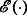denotes the Doléans-Dade exponential. We first have the following result.

### Proposition 3.4

(i) The processes $$\hat {Z}^{\mathbb {F}} X$$ and $$\hat {Z}^{\mathbb {F}}V(x,\pi)$$ are $$\mathbb {F}$$-local martingales for any portfolio (x,π) such that $$\pi \in \mathcal {A}_{\mathbb {F}}(x)$$.

(ii) The processes $$\hat {Z}^{\mathbb {G}} X$$ and $$\hat {Z}^{\mathbb {G}}V(x,\pi)$$ are $$\mathbb {G}$$-local martingales for any portfolio (x,π) such that $$\pi \in \mathcal {A}_{\mathbb {G}}(x)$$.

### Proof

We only prove assertion (ii). The same arguments can be applied to prove (i) by taking μ(G)≡0. From Itô’s formula, we have

$$d\left(\hat{Z}^{\mathbb{G}}X\right) = X_{-}d\hat{Z}^{\mathbb{G}}+ \hat{Z}_{-}^{\mathbb{G}} dX +d \left\langle{Z}^{\mathbb{G}}, X \right\rangle + d \left(\left[ Z^{\mathbb{G}}, X\right]- \left\langle{Z}^{\mathbb{G}}, X \right\rangle \right)\;.$$

From the dynamics of $$\hat {Z}^{\mathbb {G}}$$ and X, we have

$$\begin{array}{ll} d \left\langle \hat{Z}_{-}^{\mathbb{G}}, X \right\rangle &= -\hat{Z}_{-}^{\mathbb{G}} \text{Diag}(X_{-})d \left\langle \int_0^{\cdot} \left(\alpha_s+\mathbb{1}_{\{\tau\leq s\}}\mu_s(G)\right)^{\top}_{s} d\widetilde{M}_s,M\right\rangle\\ &=-\hat{Z}_{-}^\mathbb{G}\text{Diag}(X_{-}) d\langle{M} \rangle \left(\alpha+\mathbb{1}_{\left[\!\left[\right.\right.\tau,+\infty\left[\!\left[\right.\right.}\mu(G)\right)\\ &=-\hat{Z}_{-}^{\mathbb{G}}\text{Diag}(X_{-}) \left(d\langle M \rangle \alpha+d \left\langle{M}-M^{\tau} \right\rangle \mu(G)\right)\;. \end{array}$$

Therefore, we get

$$d \left(\hat{Z}^{\mathbb{G}} X\right) = X_{-}d\hat{Z}^{\mathbb{G}}+\hat Z^{\mathbb{G}}_{-} \text{Diag}(X_{-}) d \widetilde{M} +d \left(\left[ \hat{Z}^{\mathbb{G}}, X\right]- \left\langle \hat{Z}^{\mathbb{G}}, X \right\rangle \right)$$

which shows that $$\hat {Z}^{\mathbb {G}} X$$ is a $$\mathbb {G}$$-local martingale. □

We are now able to compute $$V_{\mathbb {F}}$$ and $$V_{\mathbb {G}}$$ and provide optimal strategies.

### Theorem 3.5

(i) An optimal strategy for the ordinary agent is given by

$$\pi^{ord}_{t} = \alpha_{t} \;,\quad t\in[0,T]\;,$$

and the maximal expected logarithmic utility is given by

$$V_{\mathbb{F}} = \mathbb{E}\left[\log V_{T}\left(x,\pi^{ord}\right)\right]~~=~~\log x +{1\over 2}\mathbb{E}\left[\int_{0}^{T}\alpha_{t}^{\top} d \langle M\rangle _{t}\alpha_{t}\right]\;.$$

(ii) An optimal strategy for the insider is given byand the maximal expected logarithmic utility is given by

$$\begin{array}{ll} V^{}_{\mathbb{G}} &= \mathbb{E}\left[\log V_{T}\left(x,\pi^{ins}\right)\right]\\ &= \log x +{1\over 2}\mathbb{E}\left[\int_{0}^{T}\alpha_{t}^{\top} d \langle M\rangle _{t}\alpha_{t}\right]+{1\over 2}\mathbb{E}\left[\int_{0}^{T}\mu_{t}(G)^{\top} d \langle M-M^{\tau} \rangle _{t}\mu_{t}(G)\right]\;. \end{array}$$

(iii) The insider’s additional expected utility is given by

$$V_{\mathbb{G}}-V_{\mathbb{F}} = {1\over 2}\mathbb{E}\left[\int_{0}^{T}\mu_{t}(G)^{\top} d \langle M-M^{\tau} \rangle _{t}\mu_{t}(G)\right]\;.$$

### Proof

We do not prove (i) since it relies on the same arguments as for (ii) with μ(G)≡0 and $$\hat {Z}^{\mathbb {F}}$$ in place of $$\hat {Z}^{\mathbb {G}}$$.

(ii) We recall that for a C1 concave function u such that its derivative u admits an inverse function I, we have

$$u(a) \leq u\big(I(b)\big)-b\big(I(b)-a \big)$$

for all $$a,b\in \mathbb {R}$$. Applying this inequality with u= log, a=V T (x,π) for $$\pi \in \mathcal {A}_{\mathbb {G}}(x)$$, and $$b=y\hat Z_{T}^{\mathbb {G}}$$ for some constant y>0, we get

$$\begin{array}{@{}rcl@{}} \text{log} V_{T}(x,\pi) &\leq \text{log} \frac{1}{y\hat{Z}_{T}^{\mathbb{G}}}- y\hat{Z}_{T}^{\mathbb{G}}\left(\frac{1}{y\hat{Z}_{T}^{\mathbb{G}}}-V_{T}(x,\pi)\right)\\ &\;\;\leq -\text{log} y -\text{log} \hat{Z}_{T}^{\mathbb{G}}-1 + y\hat{Z}_{T}^{G} V_{T}(x,\pi).\\ \end{array}$$

Since V(x,π) is a non-negative process and $$\hat {Z}^{\mathbb {G}} V(x,\pi)$$ is a $$\mathbb {G}$$-local martingale, it is a $$\mathbb {G}$$-super-martingale. Therefore, we get

$$\mathbb{E}\text{log} V_{T}(x,\pi) \leq -1-\text{log} y -\mathbb{E}\text{log} \hat{Z}_{T}^{\mathbb{G}} +xy\;.$$

Since this inequality holds for any $$\pi \in \mathcal {A}_{\mathbb {G}}(x)$$, we obtain by taking $$y={1\over x}$$,

$$V_{\mathbb{G}} \leq \text{log} x -\mathbb{E}\text{log} \hat{Z}_{T}^{\mathbb{G}}\;.$$

Moreover, we haveFrom 3.1 and Assumption 3.3, we get $$\pi ^{ins}\in \mathcal {A}^{}_{\mathbb {G}}(x)$$. Therefore, πins is an optimal strategy for the insider’s problem.

Using 3.1, we get that $$\int _{0}^. \alpha ^{\top } d M$$ and $$\int _{0}^. \alpha ^{\top } d \widetilde {M}$$ are, respectively, $$\mathbb {F}$$ and $$\mathbb {G}$$ martingales. Therefore, we havewhich gives

\begin{aligned} \mathbb{E}\left[\text{log} V_{T}\left(x,\pi^{ins}\right)\right] & = \text{log } x +\frac{1}{2}\mathbb{E}\left[\int_{0}^{T}\alpha_{t}^{\top} d \langle M\rangle _{t}\alpha_{t}\right]\\ &\quad +\frac{1}{2}\mathbb{E}\left[\int_{0}^{T}\mu_{t}(G)^{\top} d \left\langle M-M^{\tau} \right\rangle _{t}\mu_{t}(G)\right]\;. \end{aligned}

(iii) The result is a consequence of (i) and (ii). □

## Example of a hybrid model

In this section, we consider an explicit example where the random default time τ is given by a hybrid model as in Campi et al. (2009) and Carr and Linetsky (2006) and the information flow G is supposed to depend on the asset values at a horizon time which is similar to Guo et al. (2009).

Let B=(B t ,t≥0) be a standard Brownian motion and $$N^P=\left (N_{t}^{P},t\geq 0\right)$$ be a Poisson process with intensity $$\lambda \in \mathbb {R}_+$$. We suppose that B and NP are independent. Let $$\mathbb {F}=(\mathcal {F}_t)_{t\geq 0}$$ be the complete and right-continuous filtration generated by the processes B and NP, where $$\mathcal {F}_t=\cap _{s>t}\sigma \left \{B_u,N_{u}^{P};u\leq s\right \}$$. We define the default time τ by a hybrid model. More precisely, consider a first asset process $$S_{t}^1=\exp \left (\sigma B_t-\frac {1}{2}\sigma ^{2} t\right)$$, where σ>0, and let $$\tau _1=\inf \left \{t>0,S_{t}^{1}\leq l\right \}$$, where l is a given constant threshold such that $$l<S_{0}^{1}$$. In a similar way, consider a second asset process $$S_{t}^2=\exp \left (\lambda t-N_{t}^{P}\right)$$ and define $$\tau _2=\inf \left \{t>0,N_{t}^P=1\right \}$$. Let the default time be given by τ=τ1τ2 which is an $$\mathbb {F}$$-stopping time with a predictable component τ1 and a totally inaccessible component τ2 (this construction is borrowed from literature such as in Campi et al. (2009) and Carr and Linetsky (2006)). Let the information flow G be given by the vector $$G=\left (S_{{T^{\prime }}}^{1}, S_{{T^{\prime }}}^{2}\right)$$, where T is a horizon time. We suppose T>T since, in practice, the settlement procedure of a default event can usually be complicated and take longer time than the investment maturity. We also note that, for such a random variable G, the density assumption holds only on the interval [0,T). This explains why mathematically we impose T to be greater than the time horizon T.

We first give the density of G which is defined in (2.4). By direct computations,where ϕ is the density function of the standard normal distribution N(0,1), i.e., $$\phi (x)=\frac {1}{\sqrt {2\pi }}e^{-\frac {x^{2}}{2}}$$. Denote by $$\tilde N^{P}$$ the compensated Poisson process defined by

$$\tilde{N}^{P}_{t} = N_{t}^P-\lambda t\;,\quad t\geq 0\;.$$

The dynamics of the assets processes are then given by

\begin{aligned} dS^{1}_{t} & = S^{1}_{t} \sigma dB_{t}\;,\\ d S^{2}_{t} & = S^{2}_{t-}\left(\left(e^{-1}-1\right)d\tilde{N}_{t}^P+e^{-1}\lambda dt\right)\;. \end{aligned}

This leads us to consider the driving martingale M defined by $$M=\left (\sigma B,\left (e^{-1}-1\right)\tilde N^{P}\right)^{\top }$$. The oblique bracket of M is then given by

$$\langle M\rangle _{t} = \left(\begin{array}{ll} \sigma^{2} t & 0\\ 0 & (e^{-1}-1)^{2}\lambda t \end{array} \right)\;,\quad t\geq 0 \;.$$

Then, we can write the dynamics of the asset processes using the notations of the previous section:

$$d S^{i}_{t} = S^{i}_{t-}\left(d M^{i}_t+\alpha^{1}_{t} d \left\langle M^{i},M^{1} \right\rangle _t+\alpha^{2}_{t} d \left\langle M^{i},M^{2} \right\rangle _{t}\right)$$

with

\begin{aligned} \alpha^{1}_{t} & = 0 \\ \alpha^{2}_{t} & = {e^{-1}\over \left(e^{-1}-1\right)^{2}} \end{aligned}

for all t≥0. We can then compute the terms m1 and m2 appearing in Lemma 3.1 and we get

\begin{aligned} m^{1}_{t}(x) & = -\frac{1}{\sigma\sqrt{T^{\prime}-t}} \frac{{\phi}^{\prime}}{\phi}\left(\frac{\text{ln}(x_1)+\frac{1}{2}\sigma^2T^{\prime}-\sigma B_t}{\sigma\sqrt{T^{\prime}-t}}\right) \\ & = \frac{\text{ln}(x_1)+\frac{1}{2}\sigma^2T^{\prime}-\sigma B_t}{\sigma^{2}(T^{\prime}-t)} \end{aligned}

and

$$m^{2}_{t}(x) = \frac{1}{\left(e^{-1}-1\right)} \left({\lambda T^{\prime}-\text{ln}(x_2)-N_{t-}^{P}\over \lambda (T^{\prime}-t)}-1\right)$$

for t≥0. Since the matrix 〈M〉 is diagonal, the process μ given by Lemma 3.2 can be taken such that μ=(m1,m2). We easily check that Assumption 3.3 is satisfied. We can then apply Theorem 3.5 to the optimization problem with maturity T and we get

• an optimal strategy for the ordinary agent given by

$$\pi^{ord}_{t} = \alpha_{t} \;,\quad t\in[0,T]\;,$$

and the maximal expected utility

$$V_{\mathbb{F}} = \text{log} x +{1\over 2}\mathbb{E}\left[\int_{0}^{T}\alpha_{t}^{\top} d \langle M\rangle _{t}\alpha_{t}\right]~~=~~\text{log } x +{e^{-1}\over \left(e^{-1}-1\right)^{2}}{\lambda T\over 4}\;,$$
• an optimal strategy for the insider given by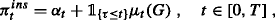and the maximal expected logarithmic utility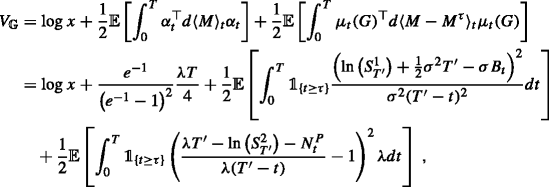• the insider’s additional expected utility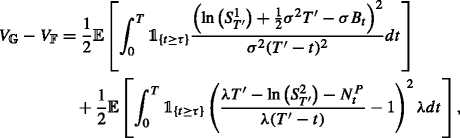whereandHence, we get

$$V_{\mathbb{G}}-V_{\mathbb{F}} = 2\mathbb{E}\left[\text{ln}\left(\frac{T^{\prime}-\tau\wedge T}{T^{\prime}-T}\right)\right].$$

We note that the gain of the insider is nonegative. In the particular case where τT, the insider has no additional information on time horizon compared to the ordinary agent. Hence their gains should be the same. This indeed holds by the previous formula since Tτ=T. In the limit case where TT, the insider may achieve a terminal wealth that is not bounded due to possible arbitrage strategies. This is also related to the condition T>T to ensure the existence of a density on [0,T] since p T explode as TT.

Concerning the optimal strategies we notice that πord is not affected by the default event, contrary to the strategy πins. The reason is the following: even the ordinary agent observes the default time which is an $$\mathbb {F}$$-stopping time, the lack of knowledge on the additional information G leads that his/her strategy remains to evolve as a strategy in the filtration $$\mathbb {F}$$ and happens to be constant over time in our simple example.

## Conclusion

We study in this paper an optimal investment problem under default risk where related information is considered as an exogenous risk added at the default time. The framework we present can also be easily adapted to information risk modelling for other sources of risks. The main contributions are twofold. First, the information flow is added at a random stopping time rather than at the initial time. Second, we consider in the optimization problem a random time which does not necessarily satisfy the standard intensity nor density hypothesis in the credit risk. From the theoretical point of view, we study the associated enlargement of filtrations and prove that Jacod’s (H’)-hypothesis holds in this setting. From the financial point of view, we obtain explicit logarithmic utility maximization results and compute the gain of the insider due to additional information.

## Appendix

### Proof

We begin with the proof of the “if” part. Assume that Z can be written in the form (2.3) such that Y is $$\mathbb {F}$$-predictable and Y(·) is $$\mathcal {P}(\mathbb {F})\otimes \mathcal {E}$$-measurable. Since τ is an $$\mathbb {F}$$-stopping time, the stochastic interval [ [0,τ] ] is a $$\mathcal {P}(\mathbb {F})$$-measurable set. Hence, the processis $$\mathbb {F}$$-predictable and hence is $$\mathbb {G}$$-predictable. It remains to prove that the processis $$\mathbb {G}$$-predictable. By a monotone class argument (see, e.g., Dellacherie and Meyer1975 Chapter I.19-24), we may assume that Y(G) is of the form Xf(G), where X is a left-continuous $$\mathbb {F}$$-adapted process, and f is a Borel function on E. Thus,is a left-continuous $$\mathbb {G}$$-adapted process, hence is $$\mathbb {G}$$-predictable. Therefore, we obtain that the process Z is $$\mathbb {G}$$-predictable.

In the following, we proceed with the proof of the “only if” part. Let Z be a $$\mathbb {G}$$-predictable process. We first show that the processis an $$\mathbb {F}$$-predictable process. Again, by a monotone class argument, we may assume that Z is left continuous. In this case the processis also left continuous. Moreover, by the left continuity of Z, one hasSince each random variable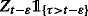is $$\mathcal {F}_{t}$$-measurable, we obtain that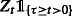is also $$\mathcal {F}_{t}$$-measurable, so that the processis $$\mathbb {F}$$-adapted and hence $$\mathbb {F}$$-predictable (since it is left continuous). Moreover, by definition one hasFor the study of the process Z on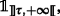we use the following characterization of the predictable σ-algebra $$\mathcal {P}(\mathbb {G})$$. The σ-algebra $$\mathcal {P}(\mathbb {G})$$ is generated by sets of the form B×[0,+) with $$B\in \mathcal {G}_{0}$$ and sets of the form B×[s,s) with 0<s<s<+ and $$B^{\prime }\in \mathcal {G}_{s-}:=\bigcup _{0\leq u<s}\mathcal {G}_{u}$$. It suffices to show that, if Z is the indicator function of such a set, then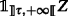can be written aswith Y(·) being a $$\mathcal {P}(\mathbb {F})\otimes \mathcal {E}$$-measurable function.

By (2.1), $$\mathcal {G}_{0}$$ is generated by $$\mathcal {F}_{0}$$ and sets of the form A∩{τ=0}, where Aσ(G). Clearly, for any $$B\in \mathcal {F}_{0}$$, the function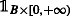is already an $$\mathbb {F}$$-predictable process. Let U be a Borel subset of E and B=G−1(U)∩{τ=0}. Let Y(·) be the $$\mathcal {P}(\mathbb {F})\otimes \mathcal {E}$$-measurable function sending $$(\omega,t,x)\in \Omega \times \mathbb {R}_{+}\times E$$ toThen, one hasBy a monotone class argument, we obtain that, if Z is of the formwith $$B\in \mathcal {G}_{0}$$, then there exists a $$\mathcal {P}(\mathbb {F})\otimes \mathcal {E}$$-measurable function Y(·) such thatIn a similar way, let s,s(0,+), s<s. By (2.1), $$\mathcal {G}_{s-}$$ is generated by $$\mathcal {F}_{s-}$$ and sets of the form A∩{τu} with u<s and Aσ(G). If $$B^{\prime }\in \mathcal {F}_{s-}$$, then the function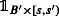is already an $$\mathbb {F}$$-predictable process. Let U be a Borel subset of E and B=G−1(U)∩{τu}. Let Y(·) be the $$\mathcal {P}(\mathbb {F})\otimes \mathcal {E}$$-measurable function sending $$(\omega,t,x)\in \Omega \times \mathbb {R}_{+}\times E$$ tothen one hasTherefore, for any process Z of the form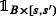with $$B\in \mathcal {F}_{s-}$$, there exists a $$\mathcal {P}(\mathbb {F})\otimes \mathcal {E}$$-measurable function Y(·) such thatThe proposition is thus proved. □

### Second proof of Theorem 2.10

The proof relies on the following Lemma, which computes the $$(\mathbb {G},\mathbb {Q})$$-predictable bracket of an $$(\mathbb {F},\mathbb {P})$$-local martingale with a general $$(\mathbb {F},\mathbb {P})$$-local martingale. This approach is more computational, but Lemma A.1 has its own interest, in particular for the study of $$\mathbb {G}$$-adapted processes. We recall that the notation I(Y(.)) has been defined in 2.6.

### Lemma A.1

Let Y be an $$\mathbb {F}$$-adapted process and Y(·) be an $$\mathbb {F}\otimes \mathcal {E}$$-adapted process such that (as in Proposition 2.6)

1. (1)

Y(x) is an $$(\mathbb {F},\mathbb {P})$$-locally square integrable martingale for any xE,

2. (2)

the process H:=I(Y(.)) is well defined and of finite variation, and-locally square-integrable martingale.

Let Z be the processThen one has

{\begin{aligned} \langle M,Z\rangle_{t}^{\mathbb{G},\mathbb{Q}}&\,=\, \left\langle M^{\tau},\widetilde{Y} \right\rangle^{\mathbb{F},\mathbb{P}}_{t}\,-\,\int_{]0,t]}M^{\tau}_{s-}\,d H_{s}\,+\,\int_{E}\left(\int_{]0,t]}U_{s-}(x)d\Lambda_{s}+\langle N,U(x)\rangle_{t}^{\mathbb{F},\mathbb{P}}\right)\eta(dx)\\ &\quad +\langle M-M^{\tau},Y(x)\rangle^{\mathbb{F},\mathbb{P}}\Big|_{x=G}, \end{aligned}}
(A.1)

where

$$U_{t}(x)=M^{\tau}_{t} Y_{t}(x)-\langle M^{\tau},Y(x)\rangle^{\mathbb{F},\mathbb{P}}_{t}+\mathbb{E}^{\mathbb{P}}\left[\mathbb{1}_{\{\tau<+\infty\}}\langle M^{\tau},Y(x)\rangle_{\tau}^{\mathbb{F},\mathbb{P}}|\mathcal{F}_{t}\right],\;x\in E.$$

### Proof

It follows from Proposition 2.6 that Z is a $$(\mathbb {G},\mathbb {Q})$$-martingale. In the following, we establish the equality (A.1).

We first treat the case where the martingale M begins at τ with M τ =0, namelyfor any t≥0. Therefore, $$W(x):=MY(x)-\langle M,Y(x)\rangle ^{\mathbb {F},\mathbb {P}}$$ is a local $$(\mathbb {F},\mathbb {P})$$-martingale which vanishes on [ [0,τ] ]. In particular, one has

$$\int_{]0,t]}W_{u-}(x)\,d\Lambda_{u}=0\quad\text{and}\quad\langle N,W(x)\rangle^{\mathbb{P},\mathbb{F}}=0$$

since both processes N and Λ are stopped at τ. By Proposition 2.6, we obtain that the processis actually a local $$(\mathbb {G},\mathbb {Q})$$-martingale. Note that

$$W(G)= MY(G)-\langle M,Y(x)\rangle^{\mathbb{F},\mathbb{P}}\big|_{x=G},$$

and $$\langle M,Y(x)\rangle ^{\mathbb {P},\mathbb {F}}\big |_{x=G}$$ is $$\mathbb {G}$$-predictable (by Proposition 2.1, we also use the fact that $$\langle M,Y(x)\rangle ^{\mathbb {P},\mathbb {F}}$$ vanishes on [ [0,τ] ]), therefore we obtain $$\langle M,Z\rangle ^{\mathbb {G},\mathbb {Q}}=\langle M,Y(x)\rangle ^{\mathbb {P},\mathbb {F}}\big |_{x=G}$$.

In the second step, we assume that M is stopped at τ. In this case, one hasand U(x) is a local $$(\mathbb {F},\mathbb {P})$$-martingale. Moreover, since M is stopped at τ, so is $$\langle M,Y(x)\rangle ^{\mathbb {F},\mathbb {P}}$$. In particular, since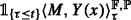is $$\mathcal {F}_{t}$$-measurable, one hasIn addition, by definition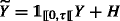Hence, one haswhere M·H and H·M denote, respectively, the integral processes

$$\int_{0}^{t}M_{s-}\,dH_{s},\quad\text{and}\quad\int_{0}^{t}H_{s-} \,dM_{s},\quad t\geq 0.$$

Since H is a predictable process of finite variation and M is an $$\mathbb {F}$$-martingale, the process [M,H] is a local $$\mathbb {F}$$-martingale (see Chapter I, Proposition 4.49 in Jacod and Shiryaev (2003)). In particular,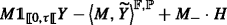is a local $$\mathbb {F}$$-martingale. Let

$$A_{t}\,=\, \left\langle M,\widetilde Y \right\rangle^{\mathbb{F},\mathbb{P}}_{t}\,-\,\int_{]0,t]}\!M_{s-}dH_{s}+\int_{E}\bigg(\int_{]0,t]}\!U_{s-}(x)\,d\Lambda_{s}\!+\langle N,U(x)\rangle_{t}^{\mathbb{F},\mathbb{P}}\bigg)\!\eta(dx),\,\, t\!\geq\! 0.$$

This is an $$\mathbb {F}$$-predictable process, and hence is $$\mathbb {G}$$-predictable. Moreover, this process is stopped at τ. Let V be the $$(\mathbb {F},\mathbb {P})$$-martingale defined as(A.2)

Note thatHence,

$$AD=VD=V_{-}\cdot D+D_{-}\cdot V+[V,D]=V_{-}\cdot N+V_{-}\cdot\Lambda+D_{-}\cdot V+[V,N]+[V,\Lambda],$$

where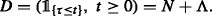In particular,

$$AD-V_{-}\cdot\Lambda-\langle V,N\rangle^{\mathbb{F},\mathbb{P}}=V_{-}\cdot N+D_{-}\cdot V+\left([V,N]-\langle V,N\rangle^{\mathbb{P},\mathbb{F}}\right)+[V,\Lambda]$$

is a local $$(\mathbb {F},\mathbb {P})$$-martingale. Therefore, one haswhich is a local $$(\mathbb {F},\mathbb {P})$$-martingale.

We write the process MZA in the form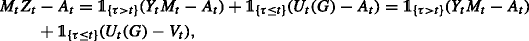where the last equality comes from (A.2). We have seen that U(x)−V is a local $$(\mathbb {F},\mathbb {P})$$-martingale for any xE. Hence, by Proposition 2.6 we obtain that MZA is a local $$(\mathbb {G},\mathbb {Q})$$-martingale.

In the final step, we consider the general case. We decompose the $$(\mathbb {F},\mathbb {P})$$-martingale into the sum of two parts Mτ and MMτ, where Mτ is an $$(\mathbb {F},\mathbb {P})$$-martingale stopped at τ, and MMτ is an $$(\mathbb {F},\mathbb {P})$$-martingale which vanishes on [ [0,τ] ]. Combining the results obtained in the two previous steps, we obtain the formula (A.1). □

Proof of Theorem 2.10. Since $$\mathbb {P}$$ and $$\mathbb {Q}$$ coincide on $$\mathbb {F}$$, we obtain that M is an $$(\mathbb {F},\mathbb {Q})$$-martingale. Moreover, since G is independent of $$\mathbb {F}$$ under the probability $$\mathbb {Q}$$, M is also a $$(\mathbb {G},\mathbb {Q})$$-martingale.

We take Z=L where we recall that $$L_{t}=\frac {d\mathbb {Q}}{d\mathbb {P}}|_{\mathcal {G}_{t}}$$ and we compute $$\langle M,Z\rangle ^{\mathbb {G},\mathbb {Q}}$$. Keeping the notation of A.1 we have

$$Y_{t}=1,\quad Y_{t}(x)=p_{t}(x),\quad t\geq 0,\;x\in E.$$

Since $$\int _{E}Y_{t}(x)\,\eta (dx)=1$$, we have

$$H_{t}=\int_{E}\left(\int_{]0,t]}Y_{u-}(x)d\Lambda_{u}+\langle N,Y(x)\rangle_{t}^{\mathbb{F},\mathbb{P}}\right)\eta(dx)=\Lambda_{t}\;,\quad t\geq 0,$$

and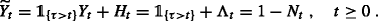Moreover, one has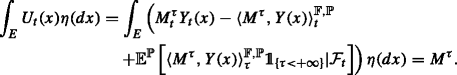Therefore, by Lemma A.1 one has

$$\begin{array}{ll} \langle M,Z\rangle^{\mathbb{G},\mathbb{Q}}&=\!-\langle M^{\tau}\!,\!N\rangle^{\mathbb{F},\mathbb{P}}\!\,-\,M_{-}^{\tau}\!\cdot\!\Lambda\,+\,M_{-}^{\tau}\!\cdot\!\Lambda\,+\,\langle M^{\tau}\!,\!N\rangle^{\mathbb{F},\mathbb{P}}\,+\,\langle M\,-\,M^{\tau}\!,\!Y(x)\rangle^{\mathbb{F},\mathbb{P}}\Big|_{x=G}\\ &=\langle M-M^{\tau},Y(x)\rangle^{\mathbb{F},\mathbb{P}}\Big|_{x=G}. \end{array}$$

Finally, since M is a $$(\mathbb {G},\mathbb {Q})$$-local martingale, by Girsanov’s theorem (cf. Jacod and Shiryaev2003 Chapter III, Theorem 3.11), the process

$$\widetilde{M}_{t}=M_{t}-\int_{]0,t]}\frac{1}{Z_{s-}}d\langle M,Z\rangle^{\mathbb{G},\mathbb{Q}}_{s},\quad t\geq 0$$

is a local $$(\mathbb {G},\mathbb {P})$$-martingale. The theorem is thus proved.

## References

• Aksamit, A, Jeanblanc, M: Enlargement of Filtration with Finance in View. SpringerBriefs in Quantitative Finance. Springer, Cham (2017).

• Amendinger, J: Martingale representation theorems for initially enlarged filtrations. Stoch. Process. Appl. 89, 101–116 (2000).

• Amendinger, J, Becherer, D, Schweizer, M: A monetary value for initial information in portfolio optimization. Finance Stochast. 7(1), 29–46 (2003).

• Amendinger, J, Imkeller, P, Schweizer, M: Additional logarithmic utility of an insider. Stoch. Process. Appl. 75, 263–286 (1998).

• Bakshi, G, Madan, D, Zhang, F: Understanding the role of recovery in default risk models: Empirical comparisons and implied recovery rates (2006). preprint, University of Maryland.

• Bielecki, TR, Rutkowski, M: Credit risk: modelling, valuation and hedging. Springer-Verlag, Berlin (2002).

• Blanchet-Scalliet, C, El Karoui, N, Jeanblanc, M, Martellini, L: Optimal investment decisions when time-horizon is uncertain. J. Math. Econ. 44(11), 1100–1113 (2008).

• Callegaro, G, Jeanblanc, M, Zargari, B: Carthaginian enlargement of filtrations. ESAIM. Probab. Stat. 17, 550–566 (2013).

• Campi, L, Polbennikov, S, A, Sbuelz: Systematic equity-based credit risk: A CEV model with jump to default. J. Econ. Dynamics Control. 33(1), 93–101 (2009).

• Carr, P, Linetsky, V: A jump to default extended CEV model: An application of Bessel processes. Finance Stochast. 10(3), 303–330 (2006).

• Dellacherie, C, Meyer, P-A: Probabilités et potentiel, Chapitres I à IV. Hermann, Paris (1975).

• Dellacherie, C, Meyer, P-A: Probabilités et potentiel. Chapitres V à VIII. Hermann, Paris (1980). Théorie des martingales.

• Duffie, D, Singleton, KJ: Credit risk: pricing, measurement and management. Princeton University Press, Princeton (2003). Princeton Series in Finance.

• Elliott, RJ, Jeanblanc, M, Yor, M: On models of default risk. Math. Finance. 10(2), 179–195 (2000).

• Föllmer, H, Imkeller, P: Anticipation cancelled by a Girsanov transformation: a paradox on Wiener space. Annales de l’Institut Henri Poincaré, Probabilités et Statistiques. 29(4), 569–586 (1993).

• Grorud, A, Pontier, M: Insider trading in a continuous time market model. Int. J. Theor. Appl. Finance. 1(3), 331–347 (1998).

• Guo, X, Jarrow, R, Zeng, Y: Modeling the recovery rate in a reduced form model. Math. Finance. 19(1), 73–97 (2009).

• Jacod, J: Grossissement initial, hypothèse (H’) et théorème de Girsanov. In: Grossissements de filtrations: exemples et applications, volume 1118 of Lecture Notes in Mathematics, pp. 15–35. Springer-Verlag, Berlin (1985).

• Jacod, J, Shiryaev, A: Limit theorems for stochastic processes, volume 288 of Grundlehren der Mathematischen Wissenschaften [Fundamental Principles of Mathematical Sciences]. second edition. Springer-Verlag, Berlin (2003).

• Jeanblanc, M, Mastrolia, T, Possamaï, D, Réveillac, A: Utility maximization with random horizon: a BSDE approach. Int. J. Theoret. Appl. Finance. 18(7), 1550045,43 (2015).

• Jeulin: Semi-martingales et grossissement d’une filtration, volume 833 of Lecture Notes in Mathematics. Springer, Berlin (1980).

• Jiao, Y, Kharroubi, I, Pham, H: Optimal investment under multiple defaults risk: a BSDE-decomposition approach. Ann. Appl. Probabil. 23(2), 455–491 (2013).

• Kchia, Y, Larsson, M, Protter, P: Linking progressive and initial filtration expansions. In: Malliavin calculus and stochastic analysis, volume 34 of Springer Proc. Math. Stat, pp. 469–487. Springer, New York (2013).

• Kchia, Y, Protter, P: On progressive filtration expansions with a process; applications to insider trading. Int. J. Theor. Appl. Finance. 18, 1550027,48 (2015).

• Kharroubi, I, Lim, T, Ngoupeyou, A: Mean-variance hedging on uncertain time horizon in a market with a jump. Appl. Math. Optimization. 68(3), 413–444 (2013).

• Lim, T, Quenez, M-C: Exponential utility maximization in an incomplete market with defaults. Electron. J. Probabil. 16(53), 1434–1464 (2011).

## Acknowledgments

The authors are grateful to the anonymous referees for their careful reading and their many insightful comments and suggestions.

## Author information

Authors

### Contributions

Both authors read and approved the final manuscript.

### Corresponding author

Correspondence to Idris Kharroubi.

## Ethics declarations

### Competing interests

The authors declare that they have no competing interests.

## Rights and permissions